GATE  >  Test: Electromagnetic Theory - 1

# Test: Electromagnetic Theory - 1

Test Description

## 20 Questions MCQ Test GATE Physics Mock Test Series | Test: Electromagnetic Theory - 1

Test: Electromagnetic Theory - 1 for GATE 2023 is part of GATE Physics Mock Test Series preparation. The Test: Electromagnetic Theory - 1 questions and answers have been prepared according to the GATE exam syllabus.The Test: Electromagnetic Theory - 1 MCQs are made for GATE 2023 Exam. Find important definitions, questions, notes, meanings, examples, exercises, MCQs and online tests for Test: Electromagnetic Theory - 1 below.
Solutions of Test: Electromagnetic Theory - 1 questions in English are available as part of our GATE Physics Mock Test Series for GATE & Test: Electromagnetic Theory - 1 solutions in Hindi for GATE Physics Mock Test Series course. Download more important topics, notes, lectures and mock test series for GATE Exam by signing up for free. Attempt Test: Electromagnetic Theory - 1 | 20 questions in 40 minutes | Mock test for GATE preparation | Free important questions MCQ to study GATE Physics Mock Test Series for GATE Exam | Download free PDF with solutions
 1 Crore+ students have signed up on EduRev. Have you?
Test: Electromagnetic Theory - 1 - Question 1

### The electric field associated with an electromagnetic wave is given by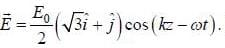If the wave propagating in fiee space, then the momentum associated with the wave is given by

Detailed Solution for Test: Electromagnetic Theory - 1 - Question 1

The intensity associated with the wave is given by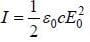Therefore, the momentum associated with the wave is given by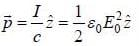Test: Electromagnetic Theory - 1 - Question 2

### Two charges +q and -q places at the points (b, 0) and (0, b) in plane as shown in figure. If the origin of the coordinates system is shifted to (b, 0), the dipole moment of the given charge distribution with respect new origin will be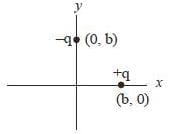Detailed Solution for Test: Electromagnetic Theory - 1 - Question 2

Total charge = -q + q = 0
Since total charge of the system is zero, so dipole moment of the system does not depends on the origin. So, if we shift the origin from (0.0) to (b. 0). The dipole moment will remain unchange.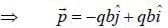Test: Electromagnetic Theory - 1 - Question 3

### Consider an infinite thin plane 3x - 4y + 2z = 6m. callying surface charge density σ. The electric E on the side of the plane containing the origin is

Detailed Solution for Test: Electromagnetic Theory - 1 - Question 3

The electric field due to infinitely plane sheet is given by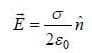The unit normal to the given plane is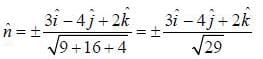There fore, the electric field on the side of the plane containing the origin is given by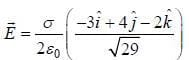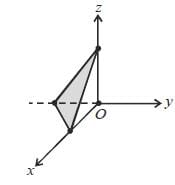Test: Electromagnetic Theory - 1 - Question 4

Consider a capacitor consisting of two fixed semicircular plates of radius R separated by a distance d. A semicircular dielectric constant k almost fills up the space between the plates and passing throughthe centre. A constant potential difference V is maintained between the plates. If the slab is now rotated throughan angle θ. then the torque acting on the slab will be

Detailed Solution for Test: Electromagnetic Theory - 1 - Question 4

The system maybe considered as the parallel combination of two capacitors c1 and cwhere. c1 and c2 are the capacitors of the parts with and without the dielectric. Thus, the equivalent capacitances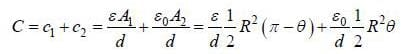Therefore, the electrostatic energy of the system is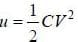Therefore. Torque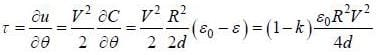Test: Electromagnetic Theory - 1 - Question 5

A filter is used to obtain nearly mnochromatic radiation from white light. If the bandwidth of filter is 8 nm and the mean wavelength is 800 nm. The coherence lenath and coherence time of the filtered liciht are respectively

Detailed Solution for Test: Electromagnetic Theory - 1 - Question 5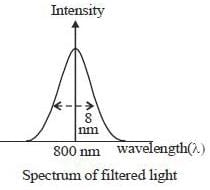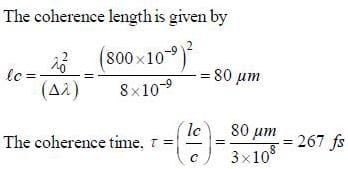*Answer can only contain numeric values
Test: Electromagnetic Theory - 1 - Question 6

Consider the propagation of EM waves dilute ionised gases such as the ionosphere. If the electron density is
1022/m3. The frequency of the wave that can passing through the gases is ____x 1010 Hz (your answer
should be an integer)

Detailed Solution for Test: Electromagnetic Theory - 1 - Question 6

We know that the ciitical frequency.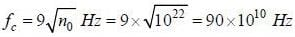*Answer can only contain numeric values
Test: Electromagnetic Theory - 1 - Question 7

In good conductorsdiffer in phase by______ degree, (answer should be an integer)

Detailed Solution for Test: Electromagnetic Theory - 1 - Question 7

In conducting medium the phase between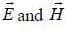is given by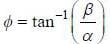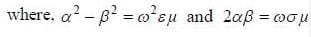For good conductors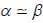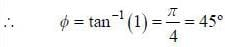*Answer can only contain numeric values
Test: Electromagnetic Theory - 1 - Question 8

A certain amount of charge Q is distributed on the circular and semicircular ring of equal radius.. If the electrostatic potential at the centre of the circle and semicircle is V1 and V2 respectively, then the ratio of V1 and V2 is ____  (answer should be an integer)

Detailed Solution for Test: Electromagnetic Theory - 1 - Question 8

For circular ring : The linear charge density on the ring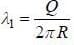Therefore, the potential at the centre of the ring is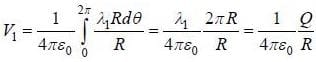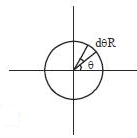For semicircular ring: The linear charge density on the semicircular ring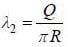Therefore, the potential at the centre of the semicircular ring is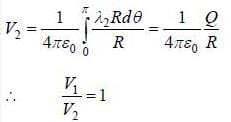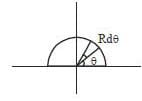*Answer can only contain numeric values
Test: Electromagnetic Theory - 1 - Question 9

An electric field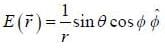exists in space. The total charge enclosed in a sphere of unit radius centred at the origin is_______ πε0. (Answer should be an integers)

Detailed Solution for Test: Electromagnetic Theory - 1 - Question 9

According to Gauss's law.
The electric flux passing through a closed surface is given by.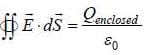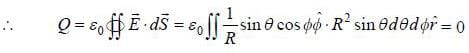*Answer can only contain numeric values
Test: Electromagnetic Theory - 1 - Question 10

A thin disk of radius R carrying uniform surface charge density σ is rotating with constant angular velocity ω about its axis (z). Suppose there is a magnetic field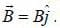The magnitude of the torque acting on the disk is ______ πσωBR4 (Upto second decimal places)

Detailed Solution for Test: Electromagnetic Theory - 1 - Question 10

Consider a ring of radius r and radial thickness dr. The change on the ring is σ2πrdr . It constitutes a current loop of current.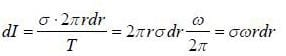The magnetic moment of this ling is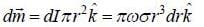Therefore, torque on the ring is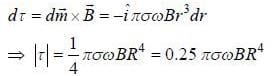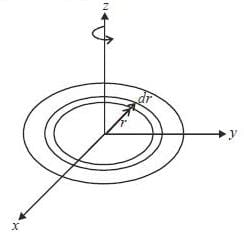Test: Electromagnetic Theory - 1 - Question 11

A conducting loop in the shape of a right angle isosceles triangle of height 10 cm is kept such that the 90° vertex is very close to an infinitely long conducting wire. The wire is electrically insulated from the loop. The hypotenuse of the triangle is parallel to the wire. The current in the triangular loop is in counterclockwise direction and increased at a constant rate of 10 As-1. Which of the following statement is true?

Detailed Solution for Test: Electromagnetic Theory - 1 - Question 11

If I current flow in the wire due to mutual induction then the magnetic field at x distance from the wire is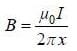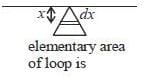Therefore, the magnetic flux passing through the loop is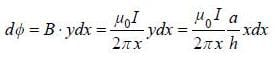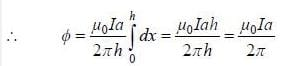Therefore, the mutual induction.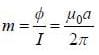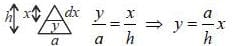Therefore, emf in the wire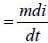(change of current in the loop)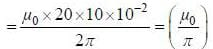Test: Electromagnetic Theory - 1 - Question 12

A rectangular loop carrying a current i is situated near a long straight wire such that the wire is parallel to one of the loop and is in the plane of the loop. If steady current I is established in the wire as shown in the figure, the loop will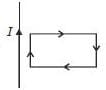Detailed Solution for Test: Electromagnetic Theory - 1 - Question 12

We know if current flow between two parallel wire in the same direction, the force will attractive and if current flow opposite direction the force will be repulsive.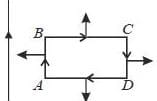As magnetic field vary with r.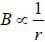So. from figure we can say there will be a net force towards the wire.

Test: Electromagnetic Theory - 1 - Question 13

Suppose a wire of radius a. length l and resistance R is carrying a steady current I with a potential difference V across the length. The poynting vector on the surface of the wire is

Detailed Solution for Test: Electromagnetic Theory - 1 - Question 13

Let's current flowing along the axis. Therefore, the electric field,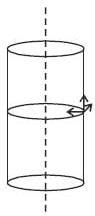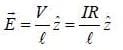According to Ampere's law.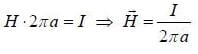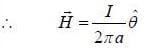Therefore, the poynting vector.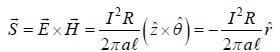Test: Electromagnetic Theory - 1 - Question 14

The electric field associated with an electromagnetic wave in certain medium is given by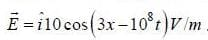The medium must be a

Detailed Solution for Test: Electromagnetic Theory - 1 - Question 14

Given: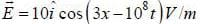Therefore, the propagating vector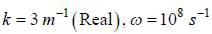Therefore, the velocity of wave in medium.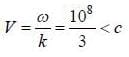Since, k is real so it can’t be lossy dielectric. Inside A perfact conductor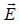will be zero.
Velocity is less than c so the medium is dielectric.

Test: Electromagnetic Theory - 1 - Question 15

Consider a waveguide of square cross-section having side a. It is to transmit an EM wave of wavelength λ. in TE10 mode but not in the TE11 or TM11 modes. The range of values of a is

Detailed Solution for Test: Electromagnetic Theory - 1 - Question 15

The cut off wave length.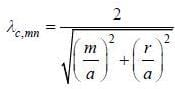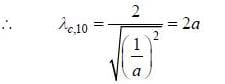So. for propagating of electro magnetic in TE10 mode its wavelength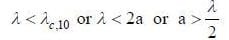And tor non-propagating ot TE11 or TM11 modes.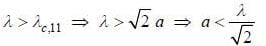Therefore, the required range of value of a is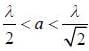Test: Electromagnetic Theory - 1 - Question 16

Suppose a particle of charge q and mass m enters with a velocity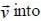a region and crossed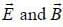fields, where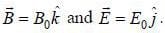If the initial velocity of the particle is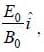then particle will follow

Detailed Solution for Test: Electromagnetic Theory - 1 - Question 16

If particle moving along x-direct ion with initia velocity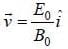Then the velocity component of the particle after enters into the region of cross electric and magnetic field is given by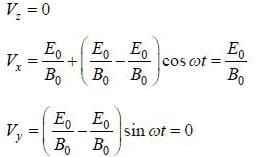Therefore, particle will moving along x-direction in a straight line path.

Test: Electromagnetic Theory - 1 - Question 17

Consider a simple magnetic field, which increases exponentially with time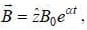where B0 and α are constant. The electric field that will be produced due to the given time varing magnetic field is

Detailed Solution for Test: Electromagnetic Theory - 1 - Question 17

According to Maxwell equation.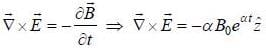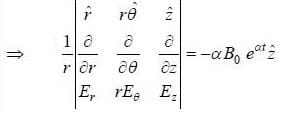Only option (c) satisfy the above equation.

Test: Electromagnetic Theory - 1 - Question 18

The E-field associated with an electromagnetic wave in from space is described by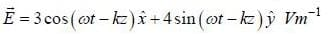The wave is incident normally on the surface (z = 0) of a perfect conductor and gets reflected. The E-field of the reflected wave tor z < 0 is

Detailed Solution for Test: Electromagnetic Theory - 1 - Question 18

Due to reflection π pliase will introduced in the E - field an d since the plane is perfectly conducting. So. amplitude will not charge.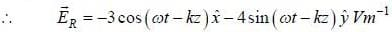*Answer can only contain numeric values
Test: Electromagnetic Theory - 1 - Question 19

A plane polarized electromagnetic wave in free space is incident normally on the surface of a conductor having complex refractive index n = 1 + ini. The phase shift of the electric vector of the reflected wave with respect to that of the incident wave is given by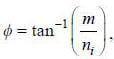the value of m is _______(answer should be integers)

Detailed Solution for Test: Electromagnetic Theory - 1 - Question 19

The reflection for normal incidence is given by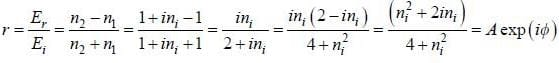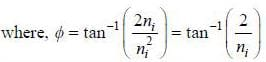*Answer can only contain numeric values
Test: Electromagnetic Theory - 1 - Question 20

Consider a waveguide of square cross-section having side a. It is to transmit an EM wave of wavelength λ. in TE10 mode but not in the TE11 or TM11 modes. The range of values of a is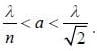The value of n is ___________(answer should be an integer)

Detailed Solution for Test: Electromagnetic Theory - 1 - Question 20

The cut off wavelength.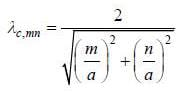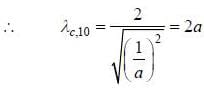So, for propagating of electro magnetic in TE10 mode its wavelength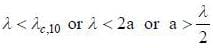And for non-propagating of TE11 or TM11 modes.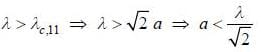Therefore, the required range of value of a is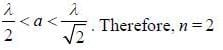## GATE Physics Mock Test Series

1 docs|34 tests
 Use Code STAYHOME200 and get INR 200 additional OFF Use Coupon Code
Information about Test: Electromagnetic Theory - 1 Page
In this test you can find the Exam questions for Test: Electromagnetic Theory - 1 solved & explained in the simplest way possible. Besides giving Questions and answers for Test: Electromagnetic Theory - 1, EduRev gives you an ample number of Online tests for practice

1 docs|34 tests